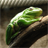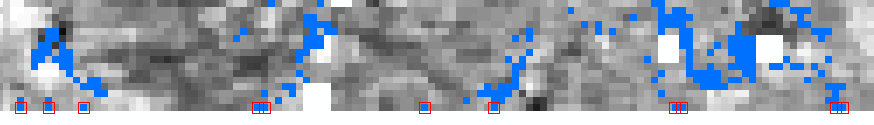# What is the proper way to do for and if statements in python?

1137
6
08-02-2017 08:55 AMNew Contributor III

I am having issues with loops and if statements in python. I keep getting an error on the last line. What am I doing wrong?

``````import arcpy
from arcpy.sa import *
from arcpy import env

sorder=r"C:\Temp\ARCGIS_PROJECT\Tutorial\Final\layers\streamorder"

desc= arcpy.Describe(sorder)

xmin= desc.extent.XMin
ymin=desc.extent.YMin
xmax= desc.extent.XMax
ymax= desc.extent.YMax
count= 0

for columns in range (xmin, xmax):
pointval= arcpy.GetCellValue_management(sorder,"%s %s" % (columns++,ymin), "1")
if (pointval== 1, pointval==2, pointval==3):
count++
print "count= %s" % (count)‍‍‍‍‍‍‍‍‍‍‍‍‍‍‍‍‍‍‍‍‍‍‍‍‍‍‍‍‍‍‍‍‍‍‍‍‍‍‍‍‍‍‍‍‍‍‍‍‍‍‍‍‍‍‍‍‍‍‍‍‍‍‍‍‍‍‍‍‍‍‍‍‍‍‍‍‍‍‍‍‍‍‍‍‍‍‍‍‍‍‍‍‍‍‍‍‍‍‍‍``````
6 RepliesFrequent Contributor

You need to have consistent spacing when indenting for and if statements.  Your for statement is indented 2 spaces, while your if statement is indented 4 spaces from the for statement when it should be 2.  Just make sure they are consistent, and that you aren't mixing spaces and tabs.

https://www.python.org/dev/peps/pep-0008/

``````for columns in range (xmin, xmax):
pointval= arcpy.GetCellValue_management(sorder,"%s %s" % (columns++,ymin), "1") #indented 2 spaces
if (pointval== 1, pointval==2, pointval==3):
count++ #indented 2 more spaces‍‍‍‍‍‍‍‍``````byEsri Esteemed Contributor

You cannot use ++ to increment a counter (this is C/C++/JS notation). In Python you need to use:

count += 1 (same for columns)byMVP Esteemed Contributor

Since you are new to Python (I assume), you may want to look at some of the links Dan Patterson‌ has /blogs/dan_patterson/2016/05/09/the-links     He has some very advanced items, but there ar also formatting tips etc.  Also, there are a few threads on geonet on "how to learn python" with many free options to get started.  Getting a few of the formatting techniques down will help a lot.  Also, there are threads on different "python IDEs"  (development environments that make it easy to see syntax error) and many of these are free too.  Just some suggestions.  Learn a bit of that and it will make the Python experience a lot of fun. (in my opinion anyway)byMVP Legendary Contributor

You shouldn't do comparisons like you are... use 'in' ... it is much cleaner, assuming that these are the only values you have.

``````pointval = 2
if pointval in (1, 2, 3):
print("yes")
else:
print("no")

yes‍‍‍‍‍‍‍‍‍‍‍‍‍``````

and you can mix object types

``````pointval = 'two'

if pointval in (1, 2, 'two', 3):
print("yes")
else:
print("no")

yes‍‍‍‍‍‍‍‍``````byEsri Esteemed Contributor

I guess that this is (any many more questions you have posted during the past days are) still related to this challenge:

Maybe it would be a lot easier to extract the last line of the raster (Extract by Rectangle—Help | ArcGIS Desktop ) and use some raster algebra (What is Map Algebra?—ArcGIS Help | ArcGIS Desktop ) to set the values that you are not interested in to NoData like:

new_raster = Con(ori_raster <= 3,  ori_raster)

... and than simply convert the raster to points: Raster to Point—Help | ArcGIS Desktop

This would result in a point featureclass with the points you are looking for.

Another method is to use convert the raster (or subset of the raster) to numpy array and read out the last line, test for the raster value and populate a point featureclass with the result.byEsri Esteemed Contributor

Below an example of using a numy array to get the values you are interested in:The blue pixel have the value we are interested in (in my case 0). The red boxes are the points that are being created by the code below. In your case line 21 would be something as proposed by Dan_Patterson: if val in (1, 2, 3):

``````import arcpy

def main():
arcpy.env.overwriteOutput = True
ras_path = r'C:\GeoNet\WUE2\r007_WUE.TIF'

# ourput featureclass
fc_out = r'C:\GeoNet\WUE2\points.shp'

# convert to numpy amd determine the sizes
ras_np = arcpy.RasterToNumPyArray(ras_path)
rows = ras_np.shape
cols = ras_np.shape

raster = arcpy.Raster(ras_path)

points = []
row = rows-1
for col in range(cols):
val = ras_np[row, col]
if int(val) == 0:
pntg = CreatePointGeometry(raster, row, col)
points.append(pntg)

arcpy.CopyFeatures_management(points, fc_out)

def CreatePointGeometry(raster, row, col):
xcell = raster.meanCellWidth
ycell = raster.meanCellHeight
xmin = raster.extent.XMin
ymax = raster.extent.YMax
sr = raster.spatialReference
pnt = arcpy.Point((col + 0.5) * xcell + xmin, ymax - (row + 0.5) * ycell)
return arcpy.PointGeometry(pnt, sr)

if __name__ == '__main__':
main()‍‍‍‍‍‍‍‍‍‍‍‍‍‍‍‍‍‍‍‍‍‍‍‍‍‍‍‍‍‍‍‍‍‍‍‍‍‍``````